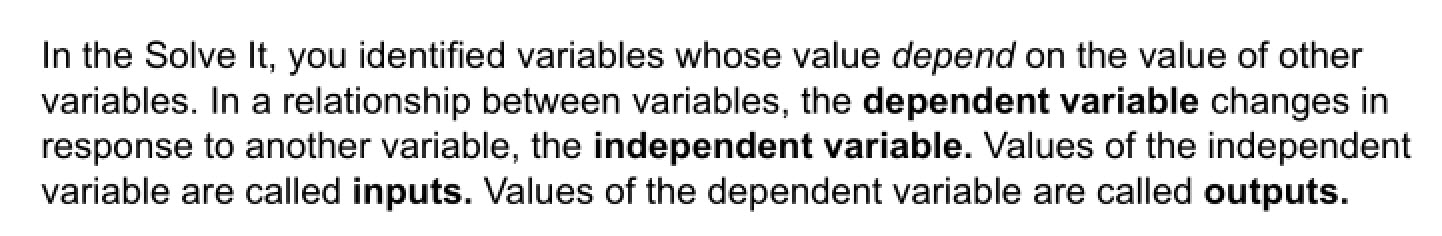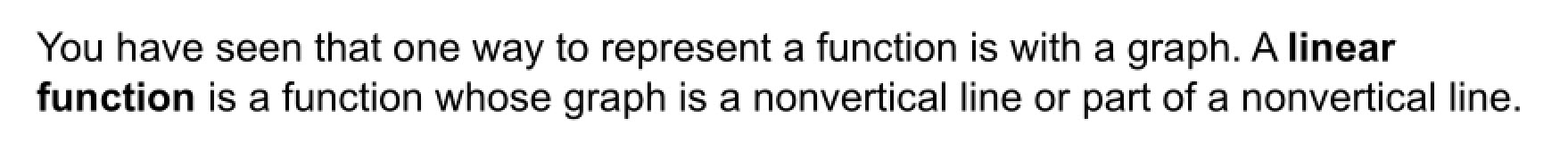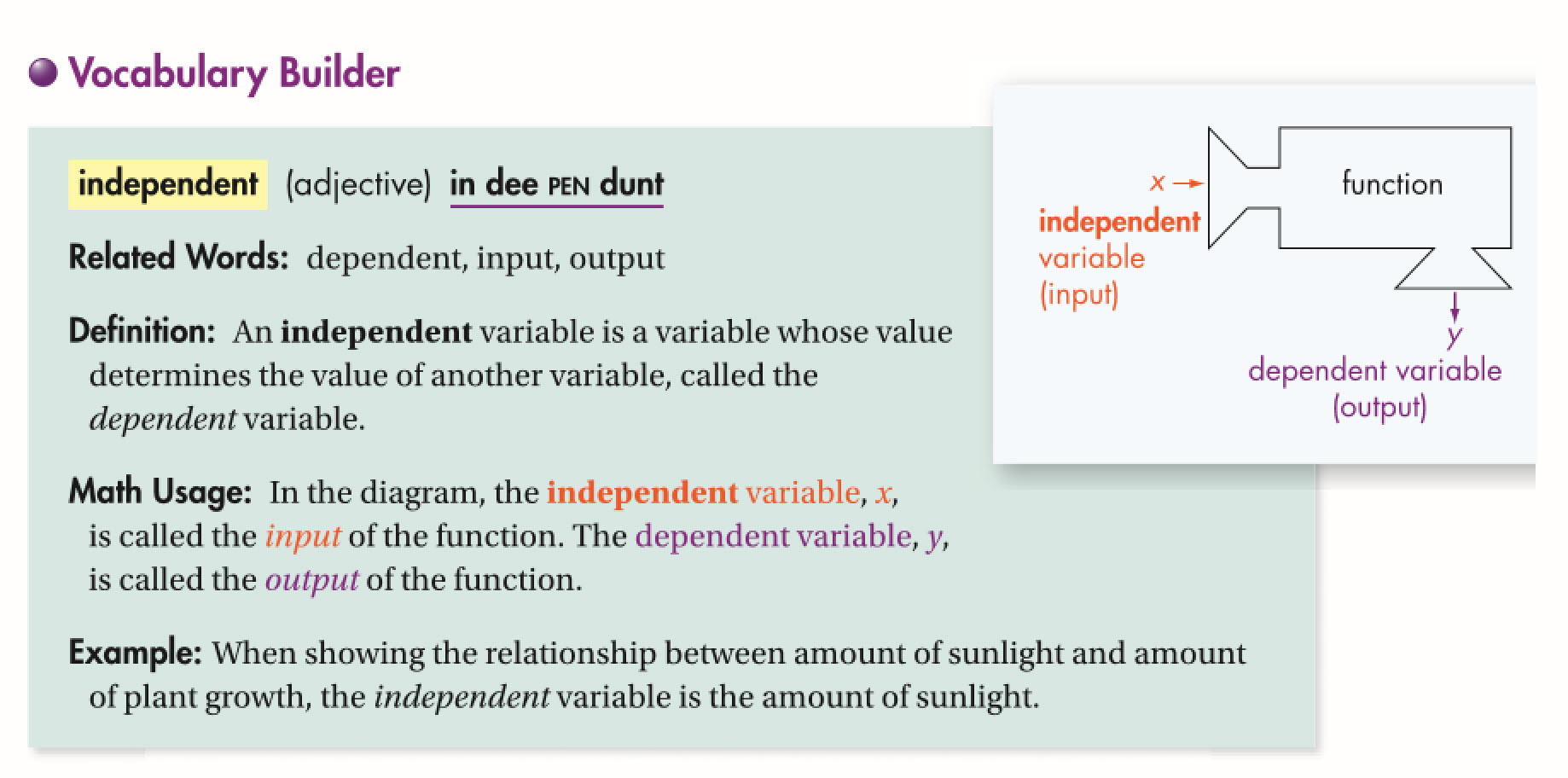Algebra 1 4-2 Patterns and Linear Functions
starstarstarstarstarstarstarstarstarstar
4.5 (1 rating)
by Matthew Richardson
| 30 Questions
Note from the author:
A complete formative lesson with embedded slideshow, mini lecture screencasts, checks for understanding, practice items, mixed review, and reflection. I create these assignments to supplement each lesson of Pearson's Common Core Edition Algebra 1, Algebra 2, and Geometry courses. See also mathquest.net and twitter.com/mathquestEDU.
The outlined content above was added from outside of Formative.1
1
10 pts
Solve It! Identify quantities in the picture that vary in response to other quantities. Select all that apply.
The amount of water that leaked from the hydrant varies depending on the amount of time it leaks
Temperature varies depending on time
The number of stop signs varies depending on the number of pigeons2
40 pts
Problem 1 Got It? Complete the items on the canvas in a color other than black.

Be sure to include relevant graph detail: label axes, indicate units on both axes, and use arrows to represent end behavior, as appropriate.
3
10 pts
Problem 1 Got It? Reasoning: Suppose you know the perimeter of n triangles. What would you do to find the perimeter of n + 1 triangles?
Add 2 to the perimeter of n triangles.
Add 3 to the perimeter of n triangles.
Add 4 to the perimeter of n triangles.
Add 5 to the perimeter of n triangles.4
40 pts
Problem 2 Got It? Complete the items on the canvas in a color other than black.
Be sure to include relevant graph detail: label axes, indicate units on both axes, and use arrows to represent end behavior, as appropriate.5
10 pts
Graph the set of ordered pairs on the same coordinate plane.
Be sure to include relevant graph detail: label axes, indicate units on both axes, and use arrows to represent end behavior, as appropriate.
7
10 pts
Graph the set of ordered pairs on the same coordinate plane.
Be sure to include relevant graph detail: label axes, indicate units on both axes, and use arrows to represent end behavior, as appropriate.
9
10 pts
Graph the set of ordered pairs on the same coordinate plane.
Be sure to include relevant graph detail: label axes, indicate units on both axes, and use arrows to represent end behavior, as appropriate.11
12
13
14
15
11
5 pts
What perimeter fills in the box?
2
4
6
8
12
5 pts
What perimeter fills in the box? Enter only the number.
13
5 pts
What perimeter fills in the box?Enter only the number.
14
5 pts
What number of squares fills in the box? Enter only the number.
15
5 pts
Write an expression to represent the perimiter in terms of the number of squares, n.
2n + 2
n + 6
4n
3n - 116
5 pts
Vocabulary: The amount of toothpaste in a tube decreases each time you brush your teeth. Identify the independent variable in this relationship.
amount of toothpaste
number of times you brush
17
5 pts
Vocabulary: The amount of toothpaste in a tube decreases each time you brush your teeth. Identify the dependent variable in this relationship.
number of times you brush
amount of toothpaste18
19
18
5 pts
Reasoning: Does set a represent a function?
Yes
No
19
10 pts
Explain.20
21
20
5 pts
Reasoning: Does set b represent a function?
Yes
No
21
10 pts
Explain.22
23
22
5 pts
Reasoning: Does set c represent a function?
Yes
No
23
10 pts
Explain.24
25
24
5 pts
Reasoning: Does the graph represent a function?
Yes
No
25
10 pts
Explain.26
27
26
10 pts
Reasoning: Does the graph represent a linear function?
Yes
No
27
5 pts
Explain.28
28
10 pts
Vocabulary Review: A function is a relationship that pairs each input value with exactly one output value. Categorize each relationship.
• Relation A
• Relation B
• Relation C
• Function
• Not a function29
10 pts
Use Your Vocabulary: Determine whether the first variable in each statement is dependent or independent.
• the growth of a plant and the light the plant receives
• the length of a pool and the time it takes to swim 1 lap
• the number of books a shelf holds and the length of the shelf
• Dependent
• Independent
30
10 pts
Reflection: Math Success